### Home > MC2 > Chapter 7 > Lesson 7.2.1 > Problem7-69

7-69.
1. Evaluate the inequality, 3x – 3 ≥ 2x + 3 , for the listed values of x. Decide if the value makes the statement true or false. Show your work. Homework Help ✎

1. x = –3

2. x = 9

3. x = 6

4.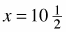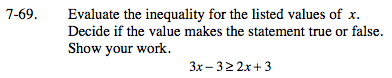Substitute each value in for x and solve the inequality to see if it is true or false.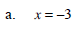3(−3) − 3 ≥ 2(−3) + 3

−9 − 3 ≥ −6 + 3

−12 ≥ −3
False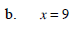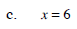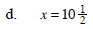True. Be sure to know why this is the answer.